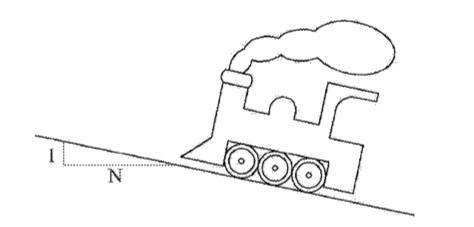# Steepest slope before slipping

• cd19

## Homework Statement

The coefficient of friction between the trains wheel's and the rail on a frosty day is 0.1, What is the steepest slope (expressed in the form ''1 in n'') that the train can climb without slipping.Ff = μ(Fn)

## The Attempt at a Solution

I have no idea. Don't want a solution, just a little guidance would be much appreciated. I understand that the point where the train will begin to slip is when the maximum friction force is reached but I can't see how to apply this to this question.

OK. You've got the force of friction.

If it's on an incline what force must it at least match in order to go uphill?

The force must be at least equal to the natural force. what I've attempted so far, I found the frictional angle to be 5.71o, by tan-1(0.1*N/N). now I'm lost.

The force must be at least equal to the natural force. what I've attempted so far, I found the frictional angle to be 5.71o, by tan-1(0.1*N/N). now I'm lost.

The statement says:
(expressed in the form ''1 in n'')

Maybe they just want it as a 1 in 10 rise or more precisely as a rise of 1 /(102 + 1)1/2 = 1 in 10.05 rise?

The statement says:

Maybe they just want it as a 1 in 10 rise or more precisely as a rise of 1 /(102 + 1)1/2 = 1 in 10.05 rise?

That would make sense, as the answer I think is 57o, but just out of curiosity how did u come to that conclusion, as it is not in the question do u just have to assume this. Freshmen to physics so excuse my ignorance if i am missing something obvious. Thanks for your help by the way.

That would make sense, as the answer I think is 57o, but just out of curiosity how did u come to that conclusion, as it is not in the question do u just have to assume this. Freshmen to physics so excuse my ignorance if i am missing something obvious. Thanks for your help by the way.

Your 5.7° answer is correct of course. I was merely noting the form that they want the answer in.

Physics is also a matter of English when it comes to supplying the answer in the right form.

So how did I come to the conclusion ...? I guess I'd say it is a careful reading of the problem. As much as anything your course may encourage you to read with precision every bit as much as understanding equations.